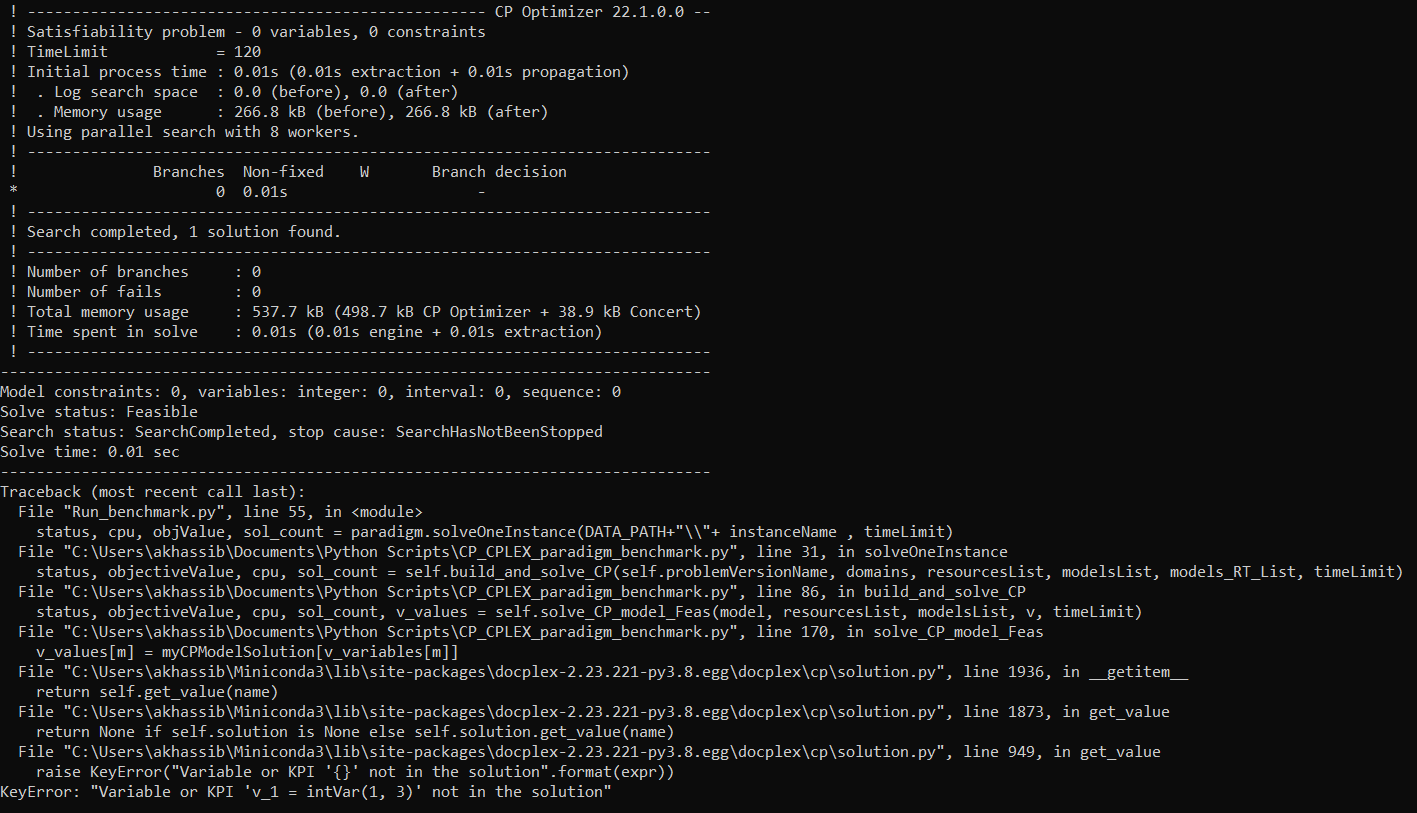# How to retrieve solution values of variables eliminated by CP Optimizer when building the model

View Only

## How to retrieve solution values of variables eliminated by CP Optimizer when building the model

Mon August 08, 2022 01:32 PM

Hello everyone,

These are my first steps with CP optimizer. I would really appreciate your help.
I have the latest version 22.10 of CPLEX.
I build and solve easy instances of resource allocation problem (resources to be assigned to tasks, according to a compatibility matrix between them). For a trivial instance with 5 resources and 1 task, I noticed that the model built is made of no variables. Consequently, retrieving the value of the single variable (v_1) of the problem is impossible:
KeyError: "Variable or KPI 'v_1 = intVar(1, 3)' not in the solution".

In this trivial instance, both 1 and 3 are solution values for v_1. However, I could not find a way to automatically retrieve any of these values using the classic `get_value`(name) from `docplex.cp.solution.``CpoSolveResult.`Turning off presolve did not help, since the variable is never added to the model. A first workaround is to add an objective minimizing v_1. However, such a workaround cannot be generalized to other cases (other versions of this problem are made of more variables, and more constraints, but still there can be some variables eliminated by CPLEX for which I was unable to retrieve solution values).

Anyone can help to force CPLEX to compute the value of these "eliminated" variables, so that I can automatize my code for all trivial and non-trivial instances?

Thank you very much for your help!

* This is how I build the CP model.

``````# create one model instance, with a name
myCPModel = CpoModel(name='Resources_Allocation_Problem')

# Variables with domains
v = {m : myCPModel.integer_var(domain= domains[m-1] , name= "v_{}".format(m)) for m in modelsList}

for m in modelsList:
print ("module m = ", m, " domain = ", domains[m-1])

myCPModel.write_information()​``````

** The output of this code snippet:
module m = 1 domain = [1, 3]
Resources = [1, 2, 3, 4, 5]
Model: Resources_Allocation_Problem
- source file: C:/Users/akhassib/Documents/Python Scripts/CP_CPLEX_paradigm_benchmark.py
- modeling time: 0.00 sec
- number of integer variables: 0
- number of interval variables: 0
- number of sequence variables: 0
- number of state functions: 0
- number of float variables: 0
- number of constraints: 0
- number of root expressions: 0
- number of expression nodes: 0
- operations: None

* This is how I solve the CP model:
``````# Solve model
myCPModelSolution = model.solve(LogVerbosity="Normal", Presolve = "On")

if myCPModelSolution:

myCPModelSolution.write()

for m in modelsList:
v_values[m] = myCPModelSolution[v[m]]
print ("v_{} = {}".format(m, v_values[m]))
``````

** The output of this code snippet:0 Favorited
0 Views
0 Files
0 Shares